# Maharashtra Board 12th Commerce Maths Solutions Chapter 2 Matrices Ex 2.2

Balbharati Maharashtra State Board 12th Commerce Maths Solution Book Pdf Chapter 2 Matrices Ex 2.2 Questions and Answers.

## Maharashtra State Board 12th Commerce Maths Solutions Chapter 2 Matrices Ex 2.2

Question 1.
If A = $$\left[\begin{array}{cc} 2 & -3 \\ 5 & -4 \\ -6 & 1 \end{array}\right]$$, B = $$\left[\begin{array}{cc} -1 & 2 \\ 2 & 2 \\ 0 & 3 \end{array}\right]$$ and C = $$\left[\begin{array}{cc} 4 & 3 \\ -1 & 4 \\ -2 & 1 \end{array}\right]$$ show that
(i) A + B = B + A
(ii) (A + B) + C = A + (B + C)
Solution: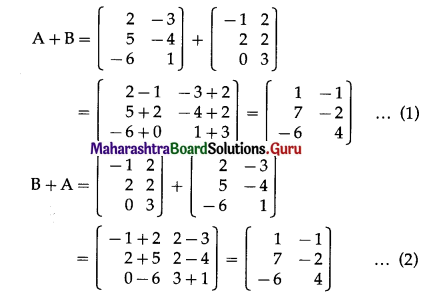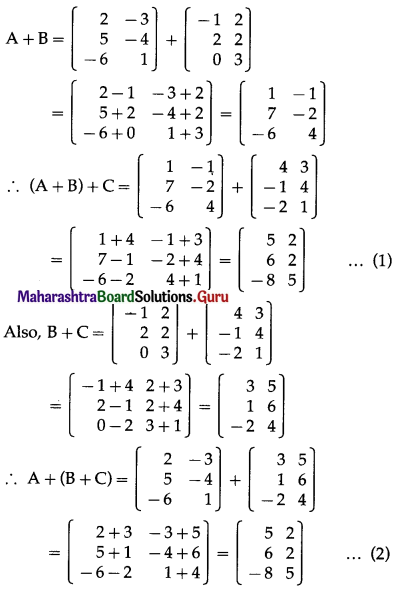From (1) and (2), we get
(A + B) + C = A + (B + C).

Question 2.
If A = $$\left[\begin{array}{cc} 1 & -2 \\ 5 & 3 \end{array}\right]$$, B = $$\left[\begin{array}{ll} 1 & -3 \\ 4 & -7 \end{array}\right]$$, then find the matrix A – 2B + 6I, where I is the unit matrix of order 2.
Solution: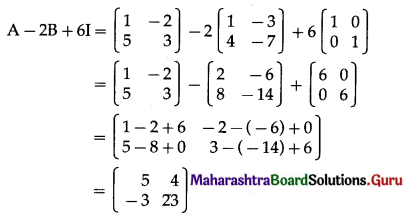Question 3.
If A = $$\left[\begin{array}{ccc} 1 & 2 & -3 \\ -3 & 7 & -8 \\ 0 & -6 & 1 \end{array}\right]$$, B = $$\left[\begin{array}{ccc} 9 & -1 & 2 \\ -4 & 2 & 5 \\ 4 & 0 & -3 \end{array}\right]$$, then find the matrix C such that A + B + C is a zero matrix.
Solution:
A + B + C = 0
∴ C = -A – B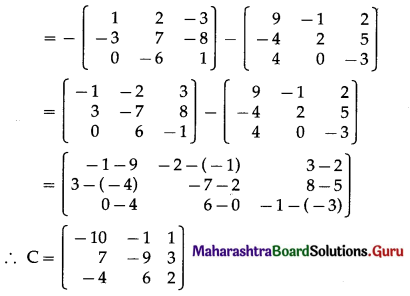Question 4.
If A = $$\left[\begin{array}{cc} 1 & -2 \\ 3 & -5 \\ -6 & 0 \end{array}\right]$$, B = $$\left[\begin{array}{cc} -1 & -2 \\ 4 & 2 \\ 1 & 5 \end{array}\right]$$ and C = $$\left[\begin{array}{cc} 2 & 4 \\ -1 & -4 \\ -3 & 6 \end{array}\right]$$, find the matrix X such that 3A – 4B + 5X = C.
Solution:
3A – 4B + 5X = C
∴ 5X = C – 3A + 4B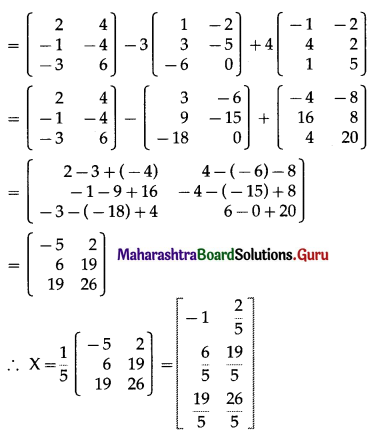Question 5.
If A = $$\left[\begin{array}{ccc} 5 & 1 & -4 \\ 3 & 2 & 0 \end{array}\right]$$, find (AT)T.
Solution:
A = $$\left[\begin{array}{ccc} 5 & 1 & -4 \\ 3 & 2 & 0 \end{array}\right]$$
∴ AT = $$\left[\begin{array}{rr} 5 & 3 \\ 1 & 2 \\ -4 & 0 \end{array}\right]$$
∴ (AT)T = $$\left[\begin{array}{ccc} 5 & 1 & -4 \\ 3 & 2 & 0 \end{array}\right]$$ = AQuestion 6.
If A = $$\left[\begin{array}{ccc} 7 & 3 & 1 \\ -2 & -4 & 1 \\ 5 & 9 & 1 \end{array}\right]$$, find (AT)T.
Solution:
A = $$\left[\begin{array}{ccc} 7 & 3 & 1 \\ -2 & -4 & 1 \\ 5 & 9 & 1 \end{array}\right]$$
∴ AT = $$\left[\begin{array}{rrr} 7 & -2 & 5 \\ 3 & -4 & 9 \\ 1 & 1 & 1 \end{array}\right]$$
∴ (AT)T = $$\left[\begin{array}{ccc} 7 & 3 & 1 \\ -2 & -4 & 1 \\ 5 & 9 & 1 \end{array}\right]$$ = A

Question 7.
Find a, b, c if $$\left[\begin{array}{ccc} 1 & \frac{3}{5} & a \\ b & -5 & -7 \\ -4 & c & 0 \end{array}\right]$$ is a symetric matrix.
Solution:
Let A = $$\left[\begin{array}{ccc} 1 & \frac{3}{5} & a \\ b & -5 & -7 \\ -4 & c & 0 \end{array}\right]$$
Since, A is a symmetric matrix, aij = aji for all i and j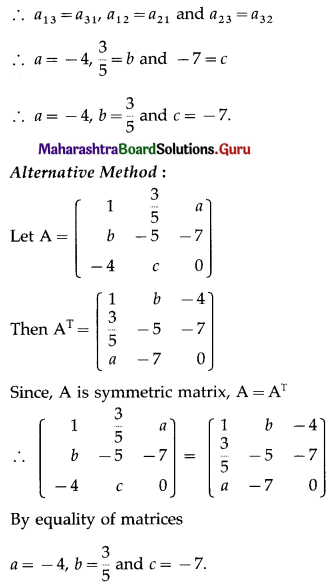Question 8.
Find x, y, z if $$\left[\begin{array}{ccc} 0 & -5 i & x \\ y & 0 & z \\ \frac{3}{2} & -\sqrt{2} & 0 \end{array}\right]$$ is a skew symmetric matrix.
Solution:
Let A = $$\left[\begin{array}{ccc} 0 & -5 i & x \\ y & 0 & z \\ \frac{3}{2} & -\sqrt{2} & 0 \end{array}\right]$$
Since, A is skew-symmetric matrix,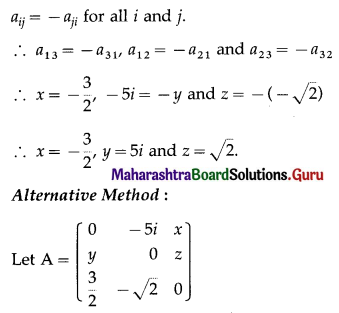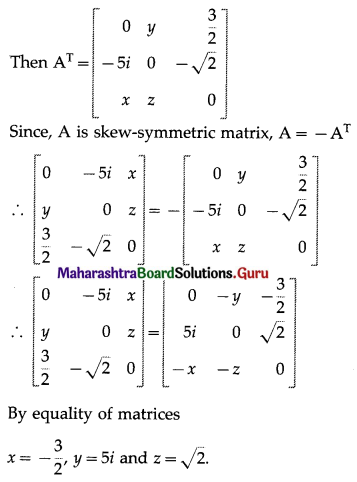Question 9.
For each of the following matrices, find its transpose and state whether it is symmetric, skew-symmetric or neither:
(i) $$\left[\begin{array}{ccc} 1 & 2 & -5 \\ 2 & -3 & 4 \\ -5 & 4 & 9 \end{array}\right]$$
Solution:
Let A = $$\left[\begin{array}{ccc} 1 & 2 & -5 \\ 2 & -3 & 4 \\ -5 & 4 & 9 \end{array}\right]$$
Then AT = $$\left[\begin{array}{rrr} 1 & 2 & -5 \\ 2 & -3 & 4 \\ -5 & 4 & 9 \end{array}\right]$$
Since, A = AT, A is a symmetric matrix.

(ii) $$\left[\begin{array}{ccc} 2 & 5 & 1 \\ -5 & 4 & 6 \\ -1 & -6 & 3 \end{array}\right]$$
Solution:
Let B = $$\left[\begin{array}{ccc} 2 & 5 & 1 \\ -5 & 4 & 6 \\ -1 & -6 & 3 \end{array}\right]$$
Then BT = $$\left(\begin{array}{rrr} 2 & -5 & -1 \\ 5 & 4 & -6 \\ 1 & 6 & 3 \end{array}\right)$$
∴ B ≠ BT
Also,
-BT = $$\left(\begin{array}{rrr} 2 & -5 & -1 \\ 5 & 4 & -6 \\ 1 & 6 & 3 \end{array}\right)=\left(\begin{array}{rrr} -2 & 5 & 1 \\ -5 & -4 & 6 \\ -1 & -6 & -3 \end{array}\right)$$
∴ B ≠ -BT
Hence, B is neither symmetric nor skew-symmetric matrix.

(iii) $$\left[\begin{array}{ccc} 0 & 1+2 i & i-2 \\ -1-2 i & 0 & -7 \\ 2-i & 7 & 0 \end{array}\right]$$
Solution: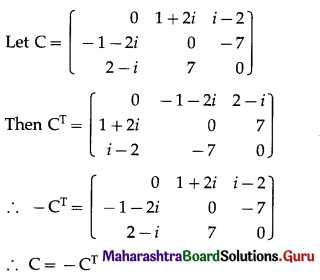Hence, C is a skew-symmetric matrix.

Question 10.
Construct the matrix A = [aij]3×3, where aij = i – j. State whether A is symmetric or skew-symmetric.
Solution: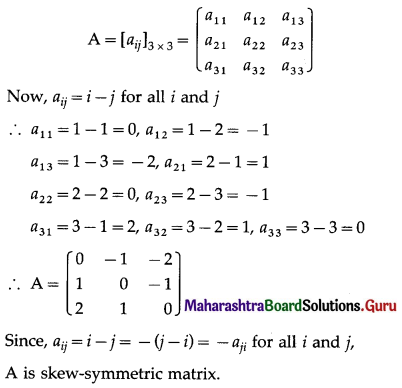Question 11.
Solve the following equations for X and Y, if 3X – Y = $$\left[\begin{array}{cc} 1 & -1 \\ -1 & 1 \end{array}\right]$$ and X – 3Y = $$\left[\begin{array}{ll} 0 & -1 \\ 0 & -1 \end{array}\right]$$
Solution: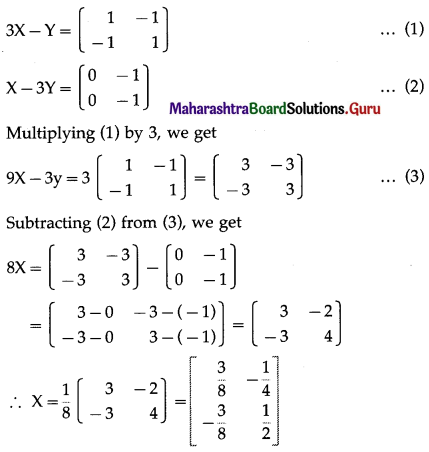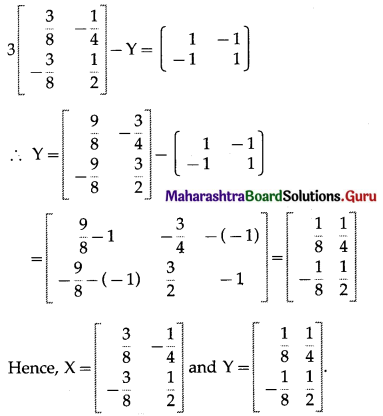Question 12.
Find matrices A and B, if 2A – B = $$\left[\begin{array}{ccc} 6 & -6 & 0 \\ -4 & 2 & 1 \end{array}\right]$$ and A – 2B = $$\left[\begin{array}{ccc} 3 & 2 & 8 \\ -2 & 1 & -7 \end{array}\right]$$
Solution: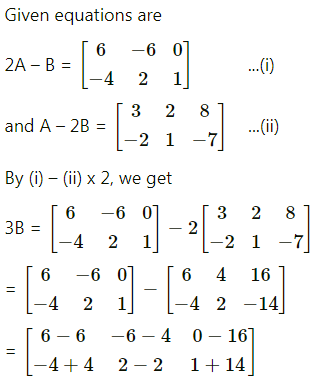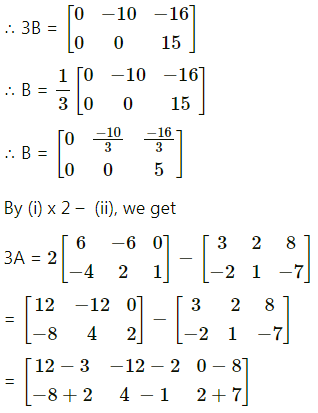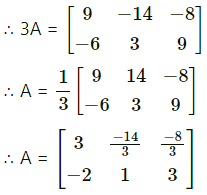Question 13.
Find x and y, if $$\left[\begin{array}{ccc} 2 x+y & -1 & 1 \\ 3 & 4 y & 4 \end{array}\right]+\left[\begin{array}{ccc} -1 & 6 & 4 \\ 3 & 0 & 3 \end{array}\right]=\left[\begin{array}{ccc} 3 & 5 & 5 \\ 6 & 18 & 7 \end{array}\right]$$
Solution: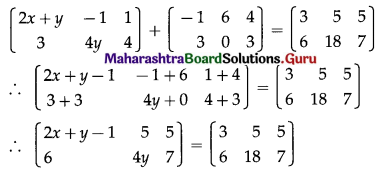By equality of matrices, we get
2x + y – 1 = 3 ……..(1)
and 4y = 18 ……….(2)
From (2), y = $$\frac{9}{2}$$
Substituting y = $$\frac{9}{2}$$ in (1), we get
2x + $$\frac{9}{2}$$ – 1 = 3
∴ 2x = 3 – $$\frac{7}{2}$$ = $$\frac{-1}{2}$$
∴ x = $$\frac{-1}{4}$$
Hence, x = $$\frac{-1}{4}$$ and y = $$\frac{9}{2}$$.Question 14.
If $$\left[\begin{array}{cc} 2 a+b & 3 a-b \\ c+2 d & 2 c-d \end{array}\right]=\left[\begin{array}{cc} 2 & 3 \\ 4 & -1 \end{array}\right]$$, find a, b, c and d.
Solution:
$$\left[\begin{array}{cc} 2 a+b & 3 a-b \\ c+2 d & 2 c-d \end{array}\right]=\left[\begin{array}{cc} 2 & 3 \\ 4 & -1 \end{array}\right]$$
By equality of matrices,
2a + b = 2 ….. (1)
3a – b = 3 …… (2)
c + 2d = 4 …… (3)
2c – d = -1 …… (4)
Adding (1) and (2), we get
5a = 5
∴ a = 1
Substituting a = 1 in (1), we get
2(1) + b = 2
∴ b = 0
Multiplying equation (4) by 2, we get
4c – 2d = -2 …… (5)
Adding (3) and (5), we get
5c = 2
∴ c = $$\frac{2}{5}$$
Substituting c = $$\frac{2}{5}$$ in (4), we get
2($$\frac{2}{5}$$) – d = -1
∴ d = $$\frac{4}{5}$$ + 1 = $$\frac{9}{5}$$
Hence, a = 1, b = 0, c = $$\frac{2}{5}$$ and d = $$\frac{9}{5}$$.Question 15.
There are two book shops owned by Suresh and Ganesh. Their sales (in Rupees) for books in three subjects – Physics, Chemistry and Mathematics for two months, July and August 2017 are given by two matrices A and B:
July sales (in Rupees), Physics, Chemistry, Mathematics
A = $$\left[\begin{array}{lll} 5600 & 6750 & 8500 \\ 6650 & 7055 & 8905 \end{array}\right]$$ First Row Suresh / Second Row Ganesh
August Sales (in Rupees), Physics, Chemistry, Mathematics
B = $$\left[\begin{array}{ccc} 6650 & 7055 & 8905 \\ 7000 & 7500 & 10200 \end{array}\right]$$ First Row Suresh / Second Row Ganesh
(i) Find the increase in sales in Z from July to August 2017.
(ii) If both book shops get 10% profit in the month of August 2017,
find the profit for each bookseller in each subject in that month.
Solution:
The sales for July and August 2017 for Suresh and Ganesh are given by the matrices A and B as: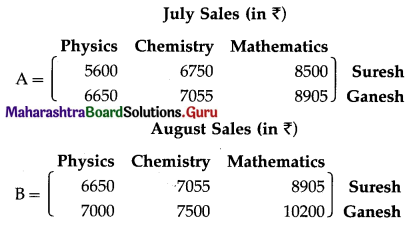(i) The increase in sales (in ₹) from July to August 2017 is obtained by subtracting the matrix A from B.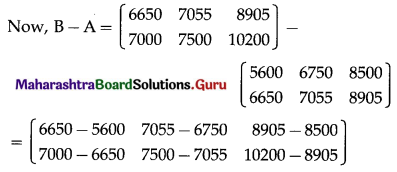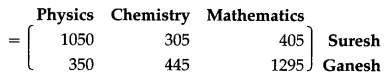Hence, the increase in sales (in ₹) from July to August 2017 for:
Suresh book shop: ₹ 1050 in Physics, ₹ 305 in Chemistry, and ₹ 405 in Mathematics.
Ganesh book shop: ₹ 350 in Physics, ₹ 445 in Chemistry, and ₹ 1295 in Mathematics.
(ii) Both the book shops get 10% profit in August 2017,
the profit for each bookseller in each subject in August 2017 is obtained by the scalar multiplication of matrix B by 10%,
i.e. $$\frac{10}{100}=\frac{1}{10}$$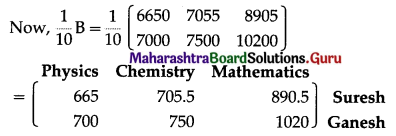Hence, the profit for Suresh book shop are ₹ 665 in Physics, ₹ 705.50 in Chemistry and ₹ 890.50 in Mathematics and for Ganesh book shop are ₹ 700 in Physics, ₹ 750 in Chemistry and ₹ 1020 in Mathematics.